Jarvis' Blog (总有美丽的风景让人流连) 总有美丽的风景让人流连

# 医学影像简介(Medical Imaging Guide)

2019-06-24
Jarvis
Post

## 1. 术语

### 1.1 切面

• 冠状面 (Coronal plane): 分离前后
• 矢状面 (Sagittal plane): 分离左右
• 横断面 (Transverse plane) / 轴向面(Axial plane): 分离上下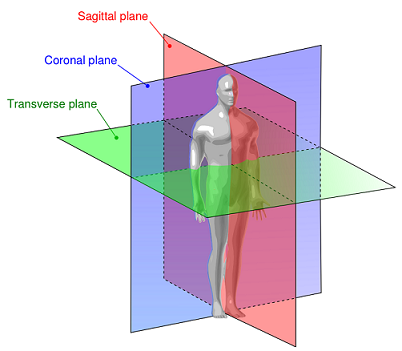### 1.2 方向

• 左 (Left)
• 右 (Right)
• 前 (Anterior)
• 后 (Posterior)
• 上 (Superior)
• 下 (Inferior)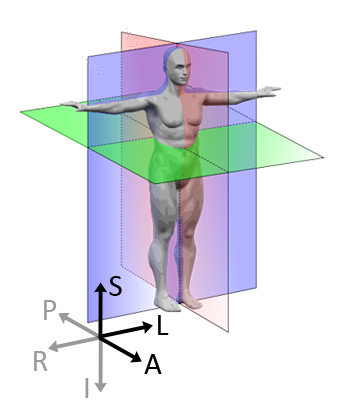### 1.3 坐标系统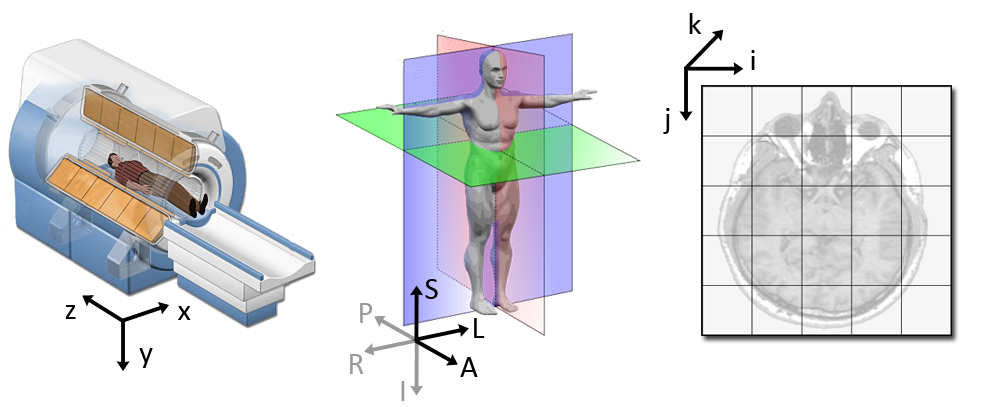#### 1.3.2 解剖坐标系(LPS/RAS/RAI)

• LPS(Left, Posterior, Superior): MHD图像(meta image), ITK工具包, ITK-Snap软件(该软件中写的是RAI, 下文会解释)使用
• RAS(Right, Anterior, Superior): Nifti图像和3D Slicer软件使用

#### 1.3.3 图像坐标系

• 原点表示图像的第一个体素在解剖坐标系中的坐标, 如(100mm, 50mm, -25mm)
• 间距表示沿着每个轴相邻体素之间的距离, 如 (1.5mm, 0.5mm, 0.5mm)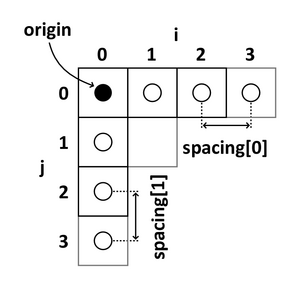#### 1.3.4 图像变换

$\left[\begin{matrix}x_1\\x_2\\x_3\end{matrix}\right]=\left[\begin{matrix}A_{11}&A_{12}&A_{13}\\A_{21}&A_{22}&A_{23}\\A_{31}&A_{32}&A_{33}\end{matrix}\right]\left[\begin{matrix}i\\j\\k\end{matrix}\right]+\left[\begin{matrix}t_1\\t_2\\t_3\end{matrix}\right].$

$\left[\begin{matrix}x_1\\x_2\\x_3\\1\end{matrix}\right]=\left[\begin{matrix}A_{11}&A_{12}&A_{13}&t_1\\A_{21}&A_{22}&A_{23}&t_2\\A_{31}&A_{32}&A_{33}&t_3\\0&0&0&1\end{matrix}\right]\left[\begin{matrix}i\\j\\k\\1\end{matrix}\right].$

#### 1.3.5 例子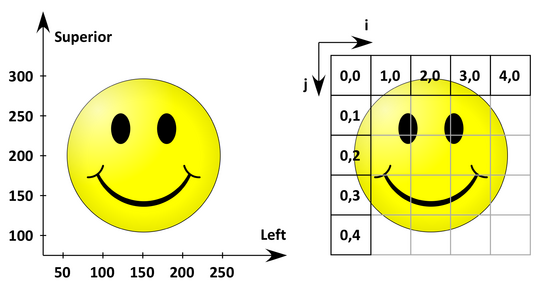$\nonumber IJtoLS = \left[\begin{matrix}50&0&50\\0&-50&300\\0&0&1\end{matrix}\right].$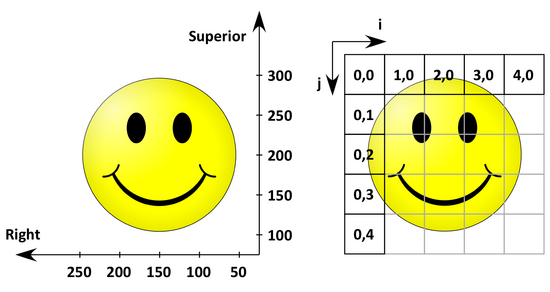$\nonumber IJtoLS = \left[\begin{matrix}-50&0&250\\0&-50&300\\0&0&1\end{matrix}\right].$

## 2. Nifti 格式 (*.nii, *.nii.gz)

Nifti 格式使用 RAS (Right, Anterior, Superior) 作为坐标系的正方向.

### 2.1 图像读取

1
2
3
import SimpleITK as sitk
arr_image = sitk.GetArrayFromImage(itk_image)


1
2
3
4
import nibabel as nib
arr_image = nib_image.get_data()


### 2.2 元信息 (meta information)

#### 2.2.1 四元组 (quaternion)

Nifti 格式的数据中使用四元组表示旋转矩阵. 从元信息中获取四元组:

1
2
3
4
import nibabel as nib


$\mathbf{R}=\left[\begin{matrix}w^2+x^2-y^2-z^2&2(xy-wz)&2(xz+wy)\\2(xy+wz)&w^2+y^2-x^2-z^2&2(yz-wx)\\2(xz-wy)&2(yz+wx)&w^2+z^2-x^2-y^2\end{matrix}\right]$

\begin{align}\nonumber &N = w^2+x^2+y^2+z^2 \\\nonumber &s=\begin{cases}0&\text{if}~N=0\\ 2/N&\text{otherwise}\end{cases} \\\nonumber \\\nonumber &wx=s\times w\times x\quad wy=s\times w\times y\quad wz=s\times w\times z \\\nonumber &xx=s\times x\times x\quad xy=s\times x\times y\quad xz=s\times x\times z \\\nonumber &yy=s\times y\times y\quad yz=s\times y\times z\quad zz=s\times z\times z \\\nonumber \\ &\mathbf{R}=\left[\begin{matrix}1-yy-zz&xy-wz&xz+wy\\xy+wz&1-xx-zz&yz-wx\\xz-wy&yz+wx&1-xx-yy\end{matrix}\right] \end{align}

#### 2.2.2 qform 仿射矩阵

• 像素间距 header['pixdim'][1:4] , 恒为正数
• 符号因子 header['pixdim'], 取值于 {-1, 1} , 其他值均视为1.
• 原点(即偏移) header['qoffset_x'], header['qoffset_y'], header['qoffset_z']

$\mathbf{Q} = \left[\begin{matrix}\mathbf{RS}&\mathbf{t}\\\mathbf{0}&1\end{matrix}\right]$

$\mathbf{S}=\left[\begin{matrix}s_1&0&0\\0&s_2&0\\0&0&qs_3\end{matrix}\right]$

#### 2.2.4 qform_code 和 sform_code

qformsform 的存在是为了更灵活的从图像坐标系到解剖坐标系的变换. Nifti 使用 qform_codesform_code 来表示选择哪种方式变换. 如下表

Name Code Description
Unknown 0 任意坐标, 使用方法1
Scanner_ANAT 1 Scanner-based anatomical coordinates.
Aligned_ANAT 2 Coordinates aligned to another file, or to the “truth” (with an arbitrary coordinate center).
Talairach 3 对齐到 Talairach 空间的坐标 (人脑的三维坐标系, 即图谱).
Mni_152 4 对齐到 mni 空间的坐标 (另一个人脑三维坐标系).

$\left[\begin{matrix}x_1\\x_2\\x_3\end{matrix}\right]=\left[\begin{matrix}i\\j\\k\end{matrix}\right]\odot\left[\begin{matrix}\text{pixdim}\\\text{pixdim}\\\text{pixdim}\end{matrix}\right].$

$\left[\begin{matrix}x_1\\x_2\\x_3\end{matrix}\right]=\mathbf{R}\left[\begin{matrix}i\\j\\qk\end{matrix}\right]\odot\left[\begin{matrix}\text{pixdim}\\\text{pixdim}\\\text{pixdim}\end{matrix}\right]+\left[\begin{matrix}\text{qoffset_x}\\\text{qoffset_y}\\\text{qoffset_z}\end{matrix}\right].$

$\left[\begin{matrix}x\\y\\z\\1\end{matrix}\right]=\left[\begin{matrix}\text{srow_x}&\text{srow_x}&\text{srow_x}&\text{srow_x}\\\text{srow_y}&\text{srow_y}&\text{srow_y}&\text{srow_y}\\\text{srow_z}&\text{srow_z}&\text{srow_z}&\text{srow_z}\\0&0&0&1\end{matrix}\right]\left[\begin{matrix}i\\j\\k\\1\end{matrix}\right].$

### 2.3 使用和查看图像

#### 2.3.1 使用 nibabel 读取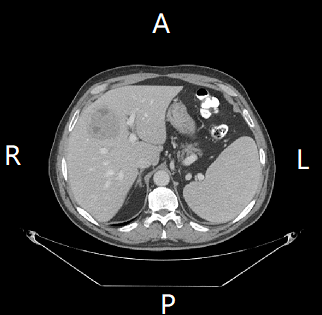• sform_code 不为0时(0表示unknown), 使用 sform , 否则
• qform_code 不为0时, 使用 qform, 否则
• 使用默认的仿射矩阵 header.get_base_affine() , 详细信息参考 nibabel 相关函数的源码

$\left[\begin{matrix}-0.97656202&0.&0.&249.1000061\\0.&0.97656202&0.&-249.0231781\\0.&0.&2.5&-651\\0.&0.&0.&1.\end{matrix}\right]$

1
2
3
4
5
6
7
8
9
10
import nibabel as nib
arr_image = nib_image.get_data()

# arr_image: [depth, height, width]
if affine[0, 0] > 0:
arr_image = numpy.flip(arr_image, axis=0)
if affine[1, 1] > 0:
arr_image = numpy.flip(arr_image, axis=1)


#### 2.3.2 使用 nibabel 保存

1
2
3
4
# 输入 Nifti1Image 的三维数组的轴应该是 (x, y, z) 的顺序, 而一般之前输出的为 (z, y, x) 的顺序, 所以需要转置一下.
out_image = np.transpose(arr_image, (2, 1, 0))
nib.save(out, out_path)


$\left[\begin{matrix}-1&0&0&0\\0&-1&0&0\\0&0&1&0\\0&0&0&1\end{matrix}\right].$

#### 2.3.2 使用 SimpleITK

1
2
3
4
5
6
7
8
import SimpleITK as sitk
arr_image = sitk.GetArrayFromImage(itk_image)
direction = itk_image.GetDirection()	# [1, 0, 0, 0, -1, 0, 0, 0, 1] reshape成3x3矩阵即可
if direction < 0:
arr_image = numpy.flip(arr_image, axis=0)
if direction < 0:
arr_image = numpy.flip(arr_image, axis=1)


## 3. Meta 格式 (*.mhd+*.raw)

### 3.1 标签 (tags)

1
2
3
4
5
6
7
8
9
10
11
12
13
ObjectType = Image
NDims = 3
BinaryData = True
BinaryDataByteOrderMSB = False
CompressedData = False
TransformMatrix = 1 0 0 0 0 -1 0 1 0
Offset = -253.125 -95 250
CenterOfRotation = 0 0 0
AnatomicalOrientation = RSA
ElementSpacing = 1.5625 1.5625 10
DimSize = 322 1078 20
ElementType = MET_SHORT
ElementDataFile = Series28.raw


• BinaryDataByteOrderMSB 中的 MSB 表示 big-endian
• TransformMatrix 为 $$3\times3$$ 的矩阵, 按列存储, 上面的一行转换为矩阵是
$\left[\begin{matrix}1&0&0\\0&0&1\\0&-1&0\end{matrix}\right]$
• Offset 为图像的第一个像素在解剖坐标系中的坐标
• CenterOfRotation 旋转中心
• AnatomicalOrientation 为解剖坐标系的From方向(关于From方向和正方向的解释参考1.3.2节). 这里做一个详细的解释:
• 该参数实际上是直接从TransformMatrix中算出来的. Meta Image默认的From方向为RAI, 与ITK相同.
• 上例中变换矩阵第三行的非零值为负数, 所以RAI的第三轴变方向, 变为RAS
• 变换矩阵第二三行交换了顺序(相对于单位阵), 所以RAS的第二三轴换序, 变为RSA
• DimSize 为图像的尺寸
• ElementType 为数据格式, 这里是 short , 即 16位有符号整数
• ElementDataFile 为数据路径
• 数据存储在 *.raw 文件中, 纯数据文件, 数据的读取的方式根据 DimSizeElementType 决定.

## Reference

• http://www.grahamwideman.com/gw/brain/orientation/orientterms.htm
• https://www.slicer.org/wiki/Coordinate_systems

• https://brainder.org/2012/09/23/the-nifti-file-format/
• https://nipy.org/nibabel/nifti_images.html

• https://itk.org/Wiki/ITK/MetaIO/Documentation
• http://www.itksnap.org/pmwiki/pmwiki.php

Content